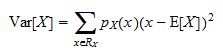StatLect

Variance formula

The variance of a random variablecan be computed using the definition of variance:wheredenotes the expected value operator.Formula for discrete variables

When the random variable is discrete the above formula becomeswhereis the set of all possible realizations ofandis the probability mass function of. In other words, we need to compute a weighted average of the squared deviations offrom its mean.

To see how to apply this formula, read some Solved exercises.

Formula for continuous variables

Whenis continuous, the formula iswhereis the probability density function of.

To see how to apply this formula, read some Solved exercises.

A simple variance formula

Instead of computing variance using these formulae, it is often easier to use the following equivalent variance formula:For example, when we know the moment generating function of, we can use it to compute the two momentsandand then plug their values in this formula.

More details

More details about this formula - as well as a proof of it and some solved exercises - can be found in the lecture entitled Variance.